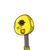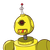# 20) If the rectangular field of perimeter 500 m has length 130 m, find itsbreadth.​

20) If the rectangular field of perimeter 500 m has length 130 m, find its

### 2 thoughts on “20) If the rectangular field of perimeter 500 m has length 130 m, find its<br />breadth.​”

1.Step-by-step explanation:

perimeter = 2(l + b)

500m = 2 ( 130+b)

500m =260+2b

500-260=2b

240/2=b

120= b.

2.